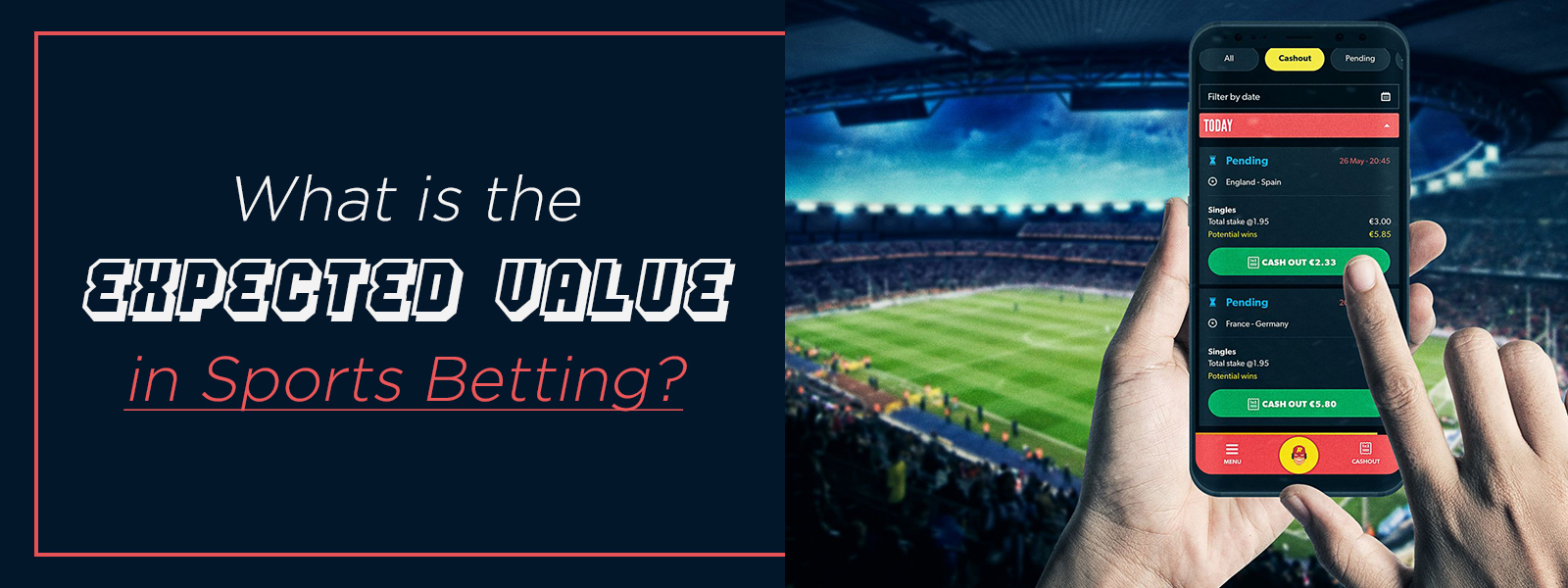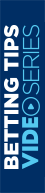What Is The Expected Value In Sports Betting?

## Expected Value In Sports Betting

People who bet on sports are to beat the bookies and win money, having fun in the process. In that sense, it is essential to know some key concepts that will lead you to obtain this objective. One of the more important is the expected value. The expected value or mathematical expectation is a mathematical term that refers to the probability that an event will occur. In sports betting, it relates to the amount that we can win or lose by placing a bet at the same odds many times. Let's look at it through the coin toss classic example. If we tossed a coin infinitely times, 50 percent of the time, it would come up heads, and the other 50 percent would be tails. In this case, the expected value would be zero since we are facing a fair game. Therefore, it is not favorable for the bookie or the bettor.

The expected value shows us how much we can expect to win on average per bet. It is the most important calculation a bettor can make to compare bookies' odds. So, how can we estimate our winnings or losses when betting on sports? We will see it below. The formula to find the mathematical expectation is simple: we multiply the winning probability by winning per bet. Then, we subtract the multiplication of the losing probability by the amount to lose per bet from this amount.

• Expected Value = (prize x winning probability) - (amount to loss x losing probability)

Let's see how to perform the calculation through an example. Suppose we plan to bet on a game between Liverpool and Watford with the following odds:

• Liverpool: 1.25
• Draw: 6.00
• Watford: 11.75

A \$ 10 bet on Watford's victory would generate\$ 117.50 revenue and a \$ 107.50 net profit. We can obtain the winning and not winning probabilities for this team by dividing one by the odds' value.

• Watford's victory probability = 1 / 11.75 = 0.075
• Watford not winning probability =
• Draw Probability + Liverpool winning Probability =
• (1 / 6.00) + (1 / 1.25) = 0.167 +0.80 = 0.967

With these data, we have that the expected value of betting on Watford's victory is:

• P = (\$ 107.5 x 0.075) - (\$ 10 x 0.967) = \$ 8.062 - \$ 9.670 = \$ - 1.608

The expected value is negative on this bet, indicating that in theory and according to the bookie's implied odds, you would lose, on average, \$ 1,608 for every \$ 10 you bet. If the result had been positive, you could hope to win money with that bet. A negative expected value does not mean that you will surely lose money. Unlike the coin toss, sports betting odds do not accurately represent a team's winning chances. So, if you can calculate some odds better than the sportsbook, you could make money. In this way, you will see where to find a positive expected value and a chance to win.

• Share:

Payment MethodJoin www.soccertipsters.com today
and start receiving our consistent soccer tips.

NEXT
Be The
Winner

Join www.soccertipsters.com today
and start receiving our consistent soccer tips.

Thank You
;